# Maharashtra Board Class 11 Physics Important Questions Chapter 13 Electromagnetic Waves and Communication System

Balbharti Maharashtra State Board 11th Physics Important Questions Chapter 13 Electromagnetic Waves and Communication System Important Questions and Answers.

## Maharashtra State Board 11th Physics Important Questions Chapter 13 Electromagnetic Waves and Communication System

Question 1.
Describe Gauss’ law of electrostatics in brief.
i. Gauss’ law of electrostatics states that electric flux through any closed surface S is equal to the total electric charge Qin enclosed by the surface divided by so.
$$\int \vec{E} \cdot \overrightarrow{\mathrm{dS}}=\frac{\mathrm{Q}_{\text {in }}}{\varepsilon_{0}}$$
where, $$\vec{E}$$ is the electric field and e0 is the permittivity of vacuum. The integral is over a closed surface S.

ii. Gauss’ law describes the relation between an electric charge and electric field it produces.

Question 2.
Describe Gauss’ law of magnetism in brief.
i. Gauss’ law for magnetism states that magnetic monopoles which are thought to be magnetic charges equivalent to the electric charges, do not exist. Magnetic poles always occur in pairs.

ii. This means, magnetic flux through a closed surface is always zero, i.e., the magnetic field lines are continuous closed curves, having neither beginning nor end.
$$\int \overrightarrow{\mathrm{B}} \cdot \overrightarrow{\mathrm{dS}}$$ = 0
where, B is the magnetic field. The integral is over a closed surface S.Question 3.
Describe Faraday’s law along with Lenz’s law.
i. Faraday’s law states that, time varying magnetic field induces an electromotive force (emf) and an electric field.

ii. Whereas, Lenz’s law states that, the direction of the induced emf is such that the change is opposed.

iii. According to Faraday’s law with Lenz’s law,
$$\int \overrightarrow{\mathrm{E}} \cdot \overrightarrow{\mathrm{d} l}=-\frac{\mathrm{d} \phi_{\mathrm{m}}}{\mathrm{dt}}$$
where, øm is the magnetic flux and the integral is over a closed loop.

Question 4.
What does Ampere’s law describe?
Ampere’s law describes the relation between the induced magnetic field associated with a loop and the current flowing through the loop.

Question 5.
Describe Ampere-Maxwell law in brief.
According to Ampere-Maxwell law, magnetic field is generated by moving charges and also by varying electric fields.
$$\int \overrightarrow{\mathrm{B}} \cdot \overrightarrow{\mathrm{d} l}=\mu_{0} \mathrm{I}+\varepsilon_{0} \mu_{0} \frac{\mathrm{d} \phi_{\mathrm{E}}}{\mathrm{dt}}$$
where, p0 and e0 are the permeability and permittivity of vacuum respectively and the integral is over a closed loop, I is the current flowing through the loop, E is the electric flux linked with the circuit.

Question 6.
What are Maxwell’s equations for charges and currents in vacuum?
$$\int \vec{E} \cdot \overrightarrow{\mathrm{dS}}=\frac{\mathrm{Q}_{\text {in }}}{\varepsilon_{0}}$$
$$\int \overrightarrow{\mathrm{B}} \cdot \overrightarrow{\mathrm{dS}}$$ = 0
$$\int \overrightarrow{\mathrm{E}} \cdot \overrightarrow{\mathrm{d} l}=-\frac{\mathrm{d} \phi_{\mathrm{m}}}{\mathrm{dt}}$$
$$\int \overrightarrow{\mathrm{B}} \cdot \overrightarrow{\mathrm{d} l}=\mu_{0} \mathrm{I}+\varepsilon_{0} \mu_{0} \frac{\mathrm{d} \phi_{\mathrm{E}}}{\mathrm{dt}}$$

Question 7.
Explain the origin of displacement current?

1. Maxwell pointed a major flaw in the Ampere’s law for time dependant fields.
2. He noticed that the magnetic field can be generated not only by electric current but also by changing electric field.
3. Therefore, he added one more term to the equation describing Ampere’s law. This term is called the displacement current.

Question 8.
In the following table, every entry on the left column can match with any number of entries on the right side. Pick up all those and write respectively against (i), (ii), and (iii).

 Name of the Physicist Work i. H. Hertz a. Existence of EM waves ii. J. Maxwell b. Properties of EM waves iii. G. Marconi c. Wireless communication d. Displacement current

(i – a, b), (ii – d), (iii – c)

Question 9.
Varying electric and magnetic fields regenerate each other. Explain.

1. According to Maxwell’s theory, accelerated charges radiate EM waves.
2. Consider a charge oscillating with some frequency. This produces an oscillating electric field in space, which produces an oscillating magnetic field which in turn is a source of oscillating electric field.
3. Thus, varying electric and magnetic fields regenerate each other.

Question 10.
Draw a neat diagram representing electromagnetic wave propagating along Z-axis.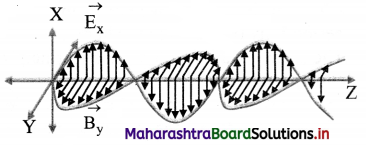Question 11.
How can energy be transported in the form of EM waves?

1. Maxwell proposed that an oscillating electric charge radiates energy in the form of EM wave.
2. EM waves are periodic changes in electric and magnetic fields, which propagate through space.
3. Thus, energy can be transported in the form of EM waves.Question 12.
State the main characteristics of EM waves.
i. The electric and magnetic fields, $$\vec{E}$$ and $$\vec{B}$$ are always perpendicular to each other and also to the direction of propagation of the EM wave. Thus, the EM waves are transverse waves.

ii. The cross product ($$\vec{E}$$ × $$\vec{B}$$) gives the direction in which the EM wave travels. ($$\vec{E}$$ × $$\vec{B}$$) also gives the energy carried by EM wave.

iii. The $$\vec{E}$$ and $$\vec{B}$$ fields vary sinusoidally and are in phase.

iv. EM waves are produced by accelerated electric charges.

v. EM waves can travel through free space as well as through solids, liquids and gases.

vi. In free space, EM waves travel with velocity c, equal to that of light in free space.
c = $$\frac {1}{\sqrt{µ_0ε_0}}$$ = 3 × 108 m/s,
where µ0 is permeability and ε0 is permittivity of free space.

vii. In a given material medium, the velocity (vm) of EM waves is given by vm = $$\frac {1}{\sqrt{µε}}$$
where µ is the permeability and ε is the permittivity of the given medium.

viii. The EM waves obey the principle of superposition.

ix. The ratio of the amplitudes of electric and magnetic fields is constant at any point and is equal to the velocity of the EM wave.
$$\left|\overrightarrow{\mathrm{E}}_{0}\right|=\mathrm{c}\left|\overrightarrow{\mathrm{B}}_{0}\right| \text { or } \frac{\left|\overrightarrow{\mathrm{E}}_{0}\right|}{\left|\overrightarrow{\mathrm{B}_{0}}\right|}=\mathrm{c}=\frac{1}{\sqrt{\mu_{0} \varepsilon_{0}}}$$
where, |$$\vec{E_0}$$| and |$$\vec{B_0}$$| are the amplitudes of $$\vec{E}$$ and $$\vec{B}$$ respectively.

x. As the electric field vector ($$\vec{E_0}$$) is more prominent than the magnetic field vector ($$\vec{B_0}$$), it is responsible for optical effects due to EM waves. For this reason, electric vector is called light vector.

xi. The intensity of a wave is proportional to the square of its amplitude and is given by the equations
$$\mathrm{I}_{\mathrm{E}}=\frac{1}{2} \varepsilon_{0} \mathrm{E}_{0}^{2}, \mathrm{I}_{\mathrm{B}}=\frac{1}{2} \frac{\mathrm{B}_{0}^{2}}{\mu_{0}}$$

xii. The energy of EM waves is distributed equally between the electric and magnetic fields. IE = IB.

Question 13.
Give reason: Electric vector is called light vector.
As the electric field vector ($$\vec{E_0}$$) is more prominent than the magnetic field vector ($$\vec{B_0}$$), it is responsible for optical effects due to EM waves. For this reason, electric vector is called light vector.

Question 14.
Explain the equations describing an EM wave.
i. In an EM wave, the magnetic field and electric field both vary sinusoidally with x.

ii. For a wave travelling along X-axis having $$\vec{E}$$ along Y-axis and $$\vec{B}$$ along the Z-axis,
Ey = E0 sin (kx – ωt)
Bz = B0 sin (kx – ωt)
where, E0 is the amplitude of the electric field (Ey) and B0 is the amplitude of the magnetic field (Bz).

iii. The propagation constant is given by k = $$\frac {2π}{λ}$$ and λ is the wavelength of the wave. The angular frequency of oscillations is given by ω = 2πv, v being the frequency of the wave.
Hence, Ey = E0 sin ($$\frac {2πx}{λ}$$ – 2πvt)
Bz = B0 sin ($$\frac {2πx}{λ}$$ – 2πvt)

iv. Both the electric and magnetic fields attain their maximum or minimum values at the same time and at the same point in space, i.e., $$\vec{E}$$ and $$\vec{B}$$ oscillate in phase with the same frequency.

Question 15.
A radio wave of frequency of 1.0 × 107 Hz propagates with speed 3 × 108 m/s. Calculate its wavelength.
Given: v= 1.0 × 107 Hz, c = 3 × 108 m/s
To find: Wavelength (λ)
Formula: vλ
Calculation: From formula,
λ = $$\frac {c}{v}$$ = $$\frac {3×10^8}{1.0×10^7}$$ = 30 m

Question 16.
A radio can tune in to any station in the 7.5 MHz to 12 MHz band. What is the corresponding wavelength band?
Given: V1 = 7.5 MHz = 7.5 × 106 Hz,
V1 = 12 MHz = 12 × 106 Hz.
To find: Wavelength band
Formula: λ = $$\frac {c}{v}$$
Calculation: From formula,
V1 = $$\frac {3×10^8}{7.5×10^6}$$ = 40 m
V1 = $$\frac {3×10^8}{12×10^6}$$ = 25 m
∴ Wavelength band = 40 m to 25 m

Question 17.
Calculate the ratio of the intensities of the two waves, if amplitude of first beam of light is 1.5 times the amplitude of second beam of light.
a1 = 1.5 a2
To find: $$\frac {I_1}{I-2}$$
Formula: I ∝ a²
Calculation: From formula,
$$\frac{I_{1}}{I_{2}}=\left(\frac{a_{1}}{a_{2}}\right)^{2}=\left(\frac{1.5 a_{2}}{a_{2}}\right)^{2}$$ = (1.5)² = 2.25

Question 18.
A beam of red light has an amplitude 2.5 times the amplitude of second beam of the same colour. Calculate the ratio of the intensities of the two waves.
a1 = 2.5 a2
To find: $$\frac {I_1}{I-2}$$
Formula: I ∝ a²
Calculation: From formula,
$$\frac{I_{1}}{I_{2}}=\left(\frac{a_{1}}{a_{2}}\right)^{2}=\left(\frac{2.5 a_{2}}{a_{2}}\right)^{2}$$ = (2.5)² = 6.25Question 19.
Calculate the velocity of EM waves in vacuum.
Given: ε0 = 8.85 × 10-12 C²/Nm²
µ0 = 4π × 10-7 Tm/A
To find: Velocity of EM waves (c)
Formula: c = $$\frac {1}{\sqrt{µ_0ε_0}}$$
Calculation: From formula,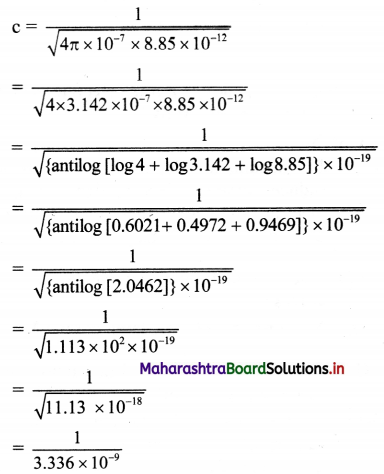………… (Taking square roots using log table)
= 0.2998 × 109 ≈ 3 × 108 m/s

Question 20.
In free space, an EM wave of frequency 28 MHz travels along the X-direction. The amplitude of the electric field is E = 9.6 V/m and its direction is along the Y-axis. What is amplitude and direction of magnetic field B?
Given: v = 28 MHz, E = 9.6 V/m,
c = 3 × 108 m/s
To find:
i. Amplitude of magnetic field (B)
ii. Direction of B
Formula:
|B| = $$\frac {|E|}{c}$$
Calculation: From formula,
|B| = $$\frac {9.6}{3×10^8}$$ = 3.2 × 10-8 T
Since that E is along Y-direction and the wave propagates along X-axis. The magnetic induction, B should be in a direction perpendicular to both X and Y axes, i.e., along the Z-direction.

Question 21.
An EM wave of frequency 50 MHz travels in vacuum along the positive X-axis and $$\vec{E}$$ at a particular point, x and at a particular instant of time t is 9.6 j V/m. Find the magnitude and direction of $$\vec{B}$$ at this point x and at instant of time t.
Given: $$\vec{E}$$ = 9.6 j V/m
i. e., Electric field E is directed along +Y axis Magnitude of $$\vec{B}$$.
|B| = $$\frac {|E|}{c}$$ = $$\frac {9.6}{3×10^8}$$ = 3.2 × 10-8 T
As the wave propagates along +X axis and E is along +Y axis, direction of B will be along +Z-axis i.e. B = 3.2 × 10-8 $$\hat{k}$$T.

Question 22.
A plane electromagnetic wave travels in vacuum along Z-direction. What can you say about the directions of its electric and magnetic field vectors? If the frequency of the wave is 30 MHz, what is its wavelength?
Since the electromagnetic waves are transverse in nature, the electric and magnetic field vectors are mutually perpendicular to each other as well as perpendicular to the direction of propagation of wave.
As the wave is travelling along Z-direction,

$$\vec{E}$$ and $$\vec{B}$$ are in XY plane.
For v = 30 MHz = 30 × 106 Hz
Wavelength, λ = $$\frac {c}{v}$$ = $$\frac {3×10^8}{30×10^6}$$ = 10 m

Question 23.
For an EM wave propagating along X direction, the magnetic field oscillates along the Z-direction at a frequency of 3 × 1010 Hz and has amplitude of 10-9 T.
i. What is the wavelength of the wave?
ii. Write the expression representing the corresponding electric field.
Given: v = 3 × 1010 Hz, B = 10-9 T
i. For wavelength of the wave:
λ = $$\frac{\mathrm{c}}{\mathrm{v}}=\frac{3 \times 10^{8}}{3 \times 10^{10}}$$ = 10-2 m

ii. Since B acts along Z-axis, E acts along Y-axis. Expression representing the oscillating electric field is
Ey = E0 sin (kx – ωt)
Ey = E0 sin [($$\frac {2π}{λ}$$)x – (2πv)t]
Ey = E0 sin 2π [$$\frac {x}{λ}$$ – vt]
Ey = E0 sin 2π [$$\frac {x}{10^{-2}}$$ – 3 × 1010 t]
Ey = E0 sin 2π [100x – 3 × 1010 t] V/m

Question 24.
The magnetic field of an EM wave travelling along X-axis is
$$\vec{B}$$ = $$\hat{k}$$ [4 × 10-4 sin (ωt – kx)]. Here B is in tesla, t is in second and x is in m. Calculate the peak value of electric force acting on a particle of charge 5 µC travelling with a velocity of 5 × 105 m/s along the Y-axis.
Expression for EM wave travelling along
X-axis, $$\vec{B}$$ = $$\hat{k}$$ [4 × 10-4 sin (ωt – kx)]
Here, B0 = 4 × 10-4
Given: q = 5 µC = 5 × 10-6 C
v = 5 × 105 m/s along Y-axis
∴ E0 = cB0 = 3 × 108 × 4 × 10-4
= 12 × 104 N/C
Maximum electric force = qE0
= 5 × 10-6 × 12 × 104
= 0.6 NQuestion 25.
The amplitude of the magnetic field part of harmonic electromagnetic wave in vaccum is B0 = 510 nT. What is the amplitude of the electric field part of the wave?
Given: B0 = 510 nT = 510 × 10-9 T
To find: Amplitude of electric field (E0)
Formula: E0 = B0C
Calculation: From formula,
E0 = 510 × 10-9 × 3 × 108
= 153V/m

Question 26.
Suppose that the electric field amplitude of an electromagnetic wave is E0 = 120 N/C and that its frequency is v = 50.0 MHz. (i) Determine, B0, ω, k, and λ. (ii) Find expressions for $$\vec{E}$$ and $$\vec{B}$$.
Solution:
For E0 = 120 N/C, v = 50 MHz = 50 × 106 Hz
i. λ = $$\frac {c}{v}$$ = $$\frac {3×10^8}{50×10^6}$$ = 6 m
B0 = $$\frac {E_0}{v}$$ = $$\frac {120}{3×10^8}$$ = 4 × 10-7 T = 400 nT
k = $$\frac {2π}{λ}$$ = $$\frac {2π}{6}$$ = 1.0472 rad/m
ω = 2πv = 2π × 50 × 106

ii. Assuming motion of em wave along X-axis, expression for electric field vector may lie along Y-axis,
∴ $$\vec{E}$$ = E0 sin (kx – ωt)
= 120 sin (1.0472 × – 3.14 × 108 t) $$\hat{j}$$ N/C
Also, magnetic field vector will lie along Z-axis, expression for magnetic field vector,
∴ $$\vec{E}$$ = B0 sin (kx – ωt)
= 4 × 10-7 sin (1.0472 × – 3.14 × 108 t) $$\hat{k}$$ T.

Question 27.
What is electromagnetic spectrum?
The orderly distribution (sequential arrangement) of EM waves according to their wavelengths (or frequencies) in the form of distinct groups having different properties is called the EM spectrum.

Question 28.
State various units used for frequency of electromagnetic waves.

1. SI unit of frequency of electromagnetic waves is hertz (Hz).
2. Higher frequencies are represented by kHz, MHz, GHz etc.
[Note: 1 kHz = 10³ Hz, 1 MHz =106 Hz. 1 GHz = 109 Hz]

Question 29.
State different units used for wavelength of electromagnetic waves.

1. The SI unit of wavelength of electromagnetic waves is metre (m).
2. Small wavelengths are represented by micrometre (µm), angstrom (Å), nanometre (nm) etc.
[Note:l A = 10-10 m = 10-8 cm, 1 µm = 10-6 m, 1 nm = 10-9 m.]

Question 30.
How are radio waves produced? State their properties and uses.
Production:

1. Radio waves are produced by accelerated motion of charges in a conducting wire. The frequency of waves produced by the circuit depends upon the magnitudes of the inductance and the capacitance.
2. Thus, by choosing suitable values of the inductance and the capacitance, radio waves of desired frequency can be produced.

Properties:

1. They have very long wavelengths ranging from a few centimetres to a few hundreds of kilometres.
2. The frequency range of AM band is 530 kHz to 1710 kHz. Frequency of the waves used for TV-transmission range from 54 MHz to 890 MHz, while those for FM radio band range from 88 MHz to 108 MHz.

Uses:

1. Radio waves are used for wireless communication purpose.
2. They are used for radio broadcasting and transmission of TV signals.
3. Cellular phones use radio waves to transmit voice communication in the ultra high frequency (UHF) band.

Question 31.
How are microwaves produced? State their properties and uses.
Production:

1. Microwaves are produced by oscillator electric circuits containing a capacitor and an inductor.
2. They can be produced by special vacuum tubes.

Properties:

1. They heat certain substances on which they are incident.
2. They can be detected by crystal detectors.

Uses:

1. Used for the transmission of TV signals.
2. Used for long distance telephone communication.
3. Microwave ovens are used for cooking.
4. Used in radar systems for the location of distant objects like ships, aeroplanes etc,
5. They are used in the study of atomic and molecular structure.

Question 32.
How are infrared waves produced? State their properties and uses.
Production:

1. All hot bodies are sources of infrared rays. About 60% of the solar radiations are infrared in nature.
2. Thermocouples, thermopile and bolometers are used to detect infrared rays.

Properties:

1. When infrared rays are incident on any object, the object gets heated.
2. These rays are strongly absorbed by glass.
3. They can penetrate through thick columns of fog, mist and cloud cover.

Uses:

1. Used in remote sensing.
2. Used in diagnosis of superficial tumours and varicose veins.
3. Used to cure infantile paralysis and to treat sprains, dislocations and fractures.
4. They are used in solar water heaters and solar cookers.
5. Special infrared photographs of the body called thermograms, can reveal diseased organs because these parts radiate less heat than the healthy organs.
6. Infrared binoculars and thermal imaging cameras are used in military applications for night vision.
7. Used to keep green house warm.
8. Used in remote controls of TV, VCR, etc.Question 33.
Write short note on visible light.

1. It is the most familiar form of EM waves.
2. These waves are detected by human eye. Therefore this wavelength range is called the visible light.
3. The visible light is emitted due to atomic excitations.
4. Visible light emitted or reflected from objects around us provides us information about those objects and hence about the surroundings.
5. Different wavelengths give rise to different colours as shown in the table given below.
 Colour Wavelength Violet 380-450 nm Blue 450-495 nm Green 495-570 nm Yellow 570-590 nm Orange 590-620 nm Red 620-750 nm

Question 34.
How are ultraviolet rays produced? State their properties and uses.
Production:

1. Ultraviolet rays can be produced by the mercury vapour lamp, electric spark and carbon arc lamp.
2. They can also be obtained by striking electrical discharge in hydrogen and xenon gas tubes.
3. The Sun is the most important natural source of ultraviolet rays, most of which are absorbed by the ozone layer in the Earth’s atmosphere.

Properties:

1. They produce fluorescence in certain materials, such as ‘phosphors’.
2. They cause photoelectric effect.
3. They cannot pass through glass but pass through quartz, fluorite, rock salt etc.
4. They possess the property of synthesizing vitamin D, when skin is exposed to them.

Uses:

1. Ultraviolet rays destroy germs and bacteria and hence they are used for sterilizing surgical instruments and for purification of water.
2. Used in burglar alarms and security systems.
3. Used to distinguish real and fake gems.
4. Used in analysis of chemical compounds.
5. Used to detect forgery.

Question 35.
How are X-rays produced? State their properties and uses.
Production:

1. German physicist W. C. Rontgen discovered X-rays while studying cathode rays. Hence, X-rays are also called Rontgen rays.
2. Cathode ray is a stream of electrons emitted by the cathode in a vacuum tube.
3. X-rays are produced when cathode rays are suddenly stopped by an obstacle.

Properties:

1. They are high energy EM waves.
2. They are not deflected by electric and magnetic fields.
3. X-rays ionize the gases through which they pass.
4. They have high penetrating power.
5. Their over dose can kill living plant and animal tissues and hence are harmful.

Uses:

1. Useful in the study of the structure of crystals.
2. X-ray photographs are useful to detect bone fracture. X-rays have many other medical uses such as CT scan.
3. X-rays are used to detect flaws or cracks in metals.
4. These are used for detection of explosives, opium etc.

Question 36.
X-rays are used in medicine and industry. Explain.
X-rays have many practical applications in medicine and industry. Because X-ray photons are of such high energy, they can penetrate several centimetres of solid matter and can be used to visualize the interiors of materials that are opaque to ordinary light.

Question 37.
How are Gamma rays produced? State their properties and uses.
Production:
Gamma rays are emitted from the nuclei of some radioactive elements such as uranium, radium etc.

Properties:

1. They are highest energy (energy range keV – GeV) EM waves.
2. They are highly penetrating.
3. They have a small ionising power.
4. They kill living cells.

Uses:

1. Used as insecticide and disinfectant for wheat and flour.
2. Used for food preservation.
3. Used in radiotherapy for the treatment of cancer and tumour.
4. They are used to produce nuclear reactions.

Question 38.
Identify the name and part of electromagnetic spectrum and arrange these wavelengths in ascending order of magnitude:
Electromagnetic waves with wavelength
i. λ1 are used by a FM radio station for broad casting.
ii. λ2 are used to detect bone fracture.
iii. λ3 are absorbed by the ozone layer of atmosphere.
iv. λ4 are used to treat muscular strain.
ii. λ2 belongs to X-rays.
iii. λ3 belongs to ultraviolet rays.
iv. λ4 belongs to infrared radiations.
Ascending order of magnitude of wavelengths:
λ3 < λ3 < λ4 < λ1

Question 39.
Explain how different types of waves emitted by stars and galaxies are observed?
i. Stars and galaxies emit different types of waves. Radio waves and visible light can pass through the Earth’s atmosphere and reach the ground without getting absorbed significantly. Thus, the radio telescopes and optical telescopes can be placed on the ground.

ii. All other type of waves get absorbed by the atmospheric gases and dust particles. Hence, the y-ray, X-ray, ultraviolet, infrared, and microwave telescopes are kept aboard artificial satellites and are operated remotely from the Earth.

iii. Even though the visible radiation reaches the surface of the Earth, its intensity decreases to some extent due to absorption and scattering by atmospheric gases and dust particles. Optical telescopes are therefore located at higher altitudes.Question 40.
In communication using radiowaves, how are EM waves propagated?
In communication using radio waves, an antenna in the transmitter radiates the EM waves, which travel through space and reach the receiving antenna at the other end.

Question 41.
Draw a schematic structure of earth’s atmosphere describing different atmospheric layers.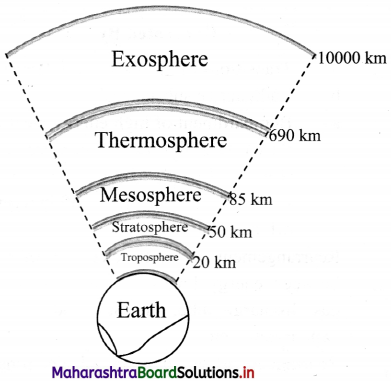Question 42.
Draw a diagram showing different types of EM waves.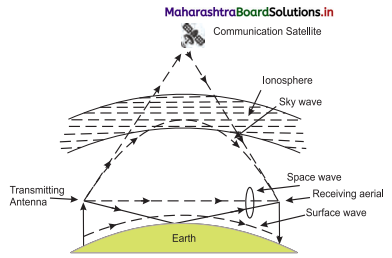Question 43.
Explain ground wave propagation.

1. When a radio wave from a transmitting antenna propagates near surface of the Earth so as to reach the receiving antenna, the wave propagation is called ground wave or surface wave propagation.
2. In this mode, radio waves travel close to the surface of the Earth and move along its curved surface from transmitter to receiver.
3. The radio waves induce currents in the ground and lose their energy by absorption. Therefore, the signal cannot be transmitted over large distances.
4. Radio waves having frequency less than 2 MHz (in the medium frequency band) are transmitted by ground wave propagation.
5. This is suitable for local broadcasting only. For TV or FM signals (very high frequency), ground wave propagation cannot be used.

Question 44.
Explain space wave propagation.
i. When the radio waves from the transmitting antenna reach the receiving antenna either directly along a straight line (line of sight) or after reflection from the ground or satellite or after reflection from troposphere, the wave propagation is called space wave propagation.

ii. The radio waves reflected from troposphere are called tropospheric waves.

iii. Radio waves with frequency greater than 30 MHz can pass through the ionosphere (60 km – 1000 km) after suffering a small deviation. Hence, these waves cannot be transmitted by space wave propagation except by using a satellite.

iv. Also, for TV signals which have high frequency, transmission over long distance is not possible by means of space wave propagation.

Question 45.
Explain the concept of range of the signal.
i. The maximum distance over which a signal can reach is called its range.

ii. For larger TV coverage, the height of the transmitting antenna should be as large as possible. This is the reason why the transmitting and receiving antennas are mounted on top of high rise buildings.

iii. Range is the straight line distance from the point of transmission (the top of the antenna) to the point on Earth where the wave will hit while travelling along a straight line.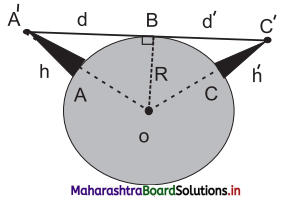iv. Let the height of the transmitting antenna (AA’) situated at A be h. B represents the point on the surface of the Earth at which the space wave hits the Earth.

v. The triangle OA’B is a right angled triangle. From ∆OA’ B,
(OA’)² = A’B² + OB²
(R + h)² = d² + R²
or R² + h² + 2Rh = d² + R² As
h << R, neglecting h²
d ≈ $$\sqrt{2Rh}$$

vi. The range can be increased by mounting the receiver at a height h’ say at a point C on the surface of the Earth. The range increases to d + d’ where d’ is 2Rh’. Thus
Total range = d + d’ = $$\sqrt{2Rh}$$ + $$\sqrt{2Rh’}$$

Question 46.
Explain sky wave propagation.1. When radio waves from a transmitting antenna reach the receiving antenna after reflection in the ionosphere, the wave propagation is called sky wave propagation.
2. The sky waves include waves of frequency between 3 MHz and 30 MHz.
3. These waves can suffer multiple reflections between the ionosphere and the Earth. Therefore, they can be transmitted over large distances.

Question 47.
What is critical frequency?
Critical frequency is the maximum value of the frequency of radio wave which can be reflected back to the Earth from the ionosphere when the waves are directed normally to ionosphere.

Question 48.
What is skip distance (zone)?
Skip distance is the shortest distance from a transmitter measured along the surface of the Earth at which a sky wave of fixed frequency (if greater than critical frequency) will be returned to the Earth so that no sky waves can be received within the skip distance.Question 49.
A radar has a power of 10 kW and is operating at a frequency of 20 GHz. It is located on the top of a hill of height 500 m. Calculate the maximum distance upto which it can detect object located on the surface of the Earth.
(Radius of Earth = 6.4 × 106 m)
Given: h = 500 m, R = 6.4 × 106 m
To find: Maximum distance or range (d)
Formula: d = $$\sqrt{2Rh}$$
Calculation: From formula,
d = $$\sqrt{2Rh}$$ = $$\sqrt{2×64×10^6×500}$$
= 8 × 104
= 80 km

Question 50.
If the height of a TV transmitting antenna is 128 m, how much square area can be covered by the transmitted signal if the receiving antenna is at the ground level? (Radius of the Earth = 6400 km)
Given: h = 128 m, R = 6400 km – 6400 × 10³ m
To find: Area covered (A)
Formulae: i. d = $$\sqrt{2Rh}$$ ii. A = πd²
Calculation:
From formula (i),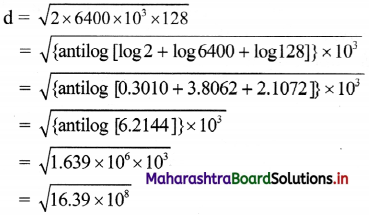= 4.048 × 104
= 40.48 km
From formula (ii).
Area covered = 3.142 × (40.48)²
= antilog [log 3.142 + 2log 40.48]
= antilog [0.4972 + 2(1.6073)]
= antilog [3.7118]
= 5.150 × 10³
= 5150 km²

Question 51.
The height of a transmitting antenna is 68 m and the receiving antenna is at the top of a tower of height 34 m. Calculate the maximum distance between them for satisfactory transmission in line of sight mode. (Radius of Earth = 6400 km)
Given: ht = 68 m, hr = 34 m,
R = 6400 km = 6.4 × 106 m
To find: Maximum distance or range (d)
Formula: d = $$\sqrt{2Rh}$$
Calculation:
From formula,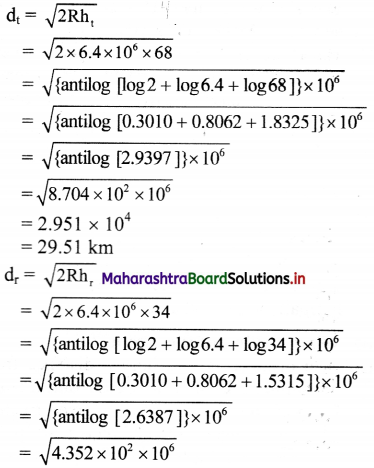= 2.086 × 104
= 20.86 km
d = dt + dr = 29.51 + 20.86 = 50.37 km

Question 52.
Explain block diagram of communication system.
i. There are three basic (essential) elements of every communication system:

1. Transmitter
2. Communication channel

ii. In a communication system, the transmitter is located at one place and the receiver at another place.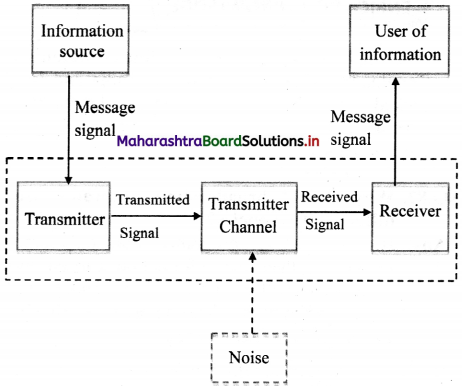iii. The communication channel is a passage through which signals transfer in between a transmitter and a receiver.

iv. This channel may be in the form of wires or cables, or may also be wireless, depending on the types of communication system.

Question 53.
What are the two different modes of communication?
i. There are two basic modes of communication:
a. point to point communication

ii. In point to point communication mode, communication takes place over a link between a single transmitter and a receiver e.g. telephony.

iii. In the broadcast mode, there are large number of receivers corresponding to the single transmitter e.g., Radio and Television transmission.Question 54.
Explain the following terms:
i. Signal
ii. Analog signal
iii. Digital signal
iv. Transmitter
v. Transducer
vii. Attenuation
viii. Amplification
ix. Range
x. Repeater
i. Signal: The information converted into electrical form that is suitable for transmission is called a signal. In a radio station, music and speech are converted into electrical form by a microphone for transmission into space. This electrical form of sound is the signal. A signal can be analog or digital.

ii. Analog signal: A continuously varying signal (voltage or current) is called an analog signal. Since a wave is a fundamental analog signal, sound and picture signals in TV are analog in nature.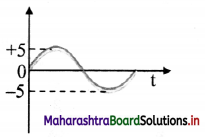iii. Digital signal: A signal (voltage or current) that can have only two discrete values is called a digital signal. For example, a square wave is a digital signal. It has two values viz, +5 V and 0 V.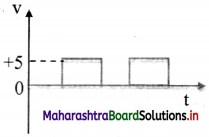iv. Transmitter: A transmitter converts the signal produced by a source of information into a form suitable for transmission through a channel and subsequent reception.

v. Transducer: A device that converts one form of energy into another form of energy is called a transducer. For example, a microphone converts sound energy into electrical energy. Therefore, a microphone is a transducer. Similarly, a loudspeaker is a transducer which converts electrical energy into sound energy.

vi. Receiver: The receiver receives the message signal at the channel output, reconstructs it in recognizable form of the original message for delivering it to the user of information.

vii. Attenuation: The loss of strength of the signal while propagating through the channel is known as attenuation. It occurs because the channel distorts, reflects and refracts the signals as it passes through it.

viii. Amplification: Amplification is the process of raising the strength of a signal, using an electronic circuit called amplifier.

ix. Range: The maximum (largest) distance between a source and a destination up to which the signal can be received with sufficient strength is termed as range.

x. Repeater: It is a combination of a transmitter and a receiver. The receiver receives the signal from the transmitter, amplifies it and transmits it to the next repeater. Repeaters are used to increase the range of a communication system.

Question 55.
Explain the role of modulation.

1. Low frequency signals cannot be transmitted over large distances. Because of this, a high frequency wave, called a carrier wave, is used.
2. Some characteristic (e.g. amplitude, frequency or phase) of this wave is changed in accordance with the amplitude of the signal. This process is known as modulation.
3. Modulation also helps avoid mixing up of signals from different transmitters as different carrier wave frequencies can be allotted to different transmitters.
4. Without the use of these waves, the audio signals, if transmitted directly by different transmitters, would have got mixed up.

Question 56.
Explain the different types of modulation.

1. Modulation can be done by modifying the amplitude (amplitude modulation), frequency (frequency modulation), and phase (phase modulation) of the carrier wave in proportion to the intensity of the signal wave keeping the other two properties same.
2. The carrier wave is a high frequency wave while the signal is a low frequency wave.
3. Waveform (a) in the figure shows a carrier wave and waveform (b) shows the signal.
4. Amplitude modulation, frequency modulation and phase modulation of carrier waves are shown in waveforms (c), (d) and (e) respectively.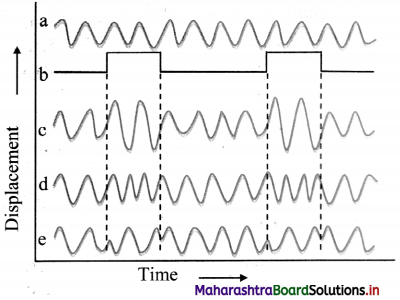Question 57.

1. It is simple to implement.
2. It has large range.
3. It is cheaper.

1. It is not very efficient as far as power usage is concerned.
2. It is prone to noise.
3. The reproduced signal may not exactly match the original signal.

In spite of this, these are used for commercial broadcasting in the long, medium and short wave bands.

Question 58.
State uses and limitations of frequency modulation.

1. Frequency modulation (FM) is more complex as compared to amplitude modulation and, therefore is more difficult to implement.
2. However, its main advantage is that it reproduces the original signal closely and is less susceptible to noise.
3. This modulation is used for high quality broadcast transmission.Question 59.
State benefits of phase modulation.

1. Phase modulation (PM) is easier than frequency modulation.
2. It is used in determining the velocity of a moving target which cannot be done using frequency modulation.

Question 60.
Given below are some famous numbers associated with electromagnetic radiations in different contexts in physics. State the part of the electromagnetic spectrum to which each belongs.
i. 21 cm (wavelength emitted by atomic hydrogen in interstellar space).
ii. 1057 MHz (frequency of radiation arising from two close energy levels in hydrogen known as Lamb Shift).
iii. 5890 A – 5896 A [double lines of sodium]
i. Radio waves (short wavelength or high frequency end)
ii. Radio waves (short wavelength or high frequency end)
iii. Visible region (yellow light)

Question 61.
Vidhya and Vijay were studying the effect of certain radiations on flower plants. Vidhya exposed her plants to UV rays and Vijay exposed his plants to infrared rays. After few days, Vidhya’s plants got damaged and Vijay’s plants had beautiful bloom. Why did this happen?
Frequency of UV rays is greater than infrared rays, hence UV rays are much more energetic than infrared rays. Plants cannot tolerate the exposure of high energy rays. As a result, Vidhya’s plants got damaged and Vijay’s plants had a beautiful bloom.

Multiple Choice Questions

Question 1.
Which of the following type of radiations are radiated by an oscillating electric charge?
(A) Electric
(B) Magnetic
(C) Thermoelectric
(D) Electromagnetic
(D) Electromagnetic

Question 2.
If $$\vec{E}$$ and $$\vec{B}$$ are the electric and magnetic field vectors of e.m. waves, then the direction of propagation of e.m. direction of wave is along the
(A) $$\vec{E}$$
(B) $$\vec{B}$$
(C) $$\vec{E}$$ × $$\vec{B}$$
(D) $$\vec{E}$$ • $$\vec{B}$$
(C) $$\vec{E}$$ × $$\vec{B}$$

Question 3.
The unit of expression µ0o ε0 is
(A) m / s
(B) m² / s²
(C) s² / m²
(D) s / m
(C) s² / m²

Question 4.
According to Maxwell’s equation the velocity of light in any medium is expressed as
(A) $$\frac {1}{\sqrt{µ_0ε_0}}$$
(B) $$\frac {22}{\sqrt{µε}}$$
(C) $$\sqrt{\frac {µ}{ε}}$$
(D) $$\sqrt{\frac {µ_0}{ε}}$$
(B) $$\frac {22}{\sqrt{µε}}$$

Question 5.
The electromagnetic waves do not transport.
(A) energy
(B) charge
(C) momentum
(D) pressure
(B) charge

Question 6.
In an electromagnetic wave, the direction of the magnetic induction $$\vec{B}$$ is
(A) parallel to the electric field $$\vec{E}$$.
(B) perpendicular to the electric field $$\vec{E}$$.
(C) antiparallel to the pointing vector $$\vec{S}$$.
(D) random.
(B) perpendicular to the electric field $$\vec{E}$$.Question 7.
Which of the following electromagnetic waves have the longest wavelength?
(A) heat waves
(B) light waves
(D) microwaves.

Question 8.
Radio waves do not penetrate in the band of
(A) ionosphere
(B) mesosphere
(C) troposphere
(D) stratosphere
(A) ionosphere

Question 9.
Which of the following electromagnetic wave has least wavelength?
(A) Gamma rays
(B) X- rays
(D) microwaves
(A) Gamma rays

Question 10.
If E is an electric field and $$\vec{B}$$ is the magnetic induction, then the energy flow per unit area per unit time in an electromagnetic field is given by
(A) $$\frac {1}{µ_0}$$ $$\vec{E}$$ × $$\vec{B}$$
(B) $$\vec{E}$$.$$\vec{B}$$
(C) E² + B²
(D) $$\frac {E}{B}$$
(A) $$\frac {1}{µ_0}$$ $$\vec{E}$$ × $$\vec{B}$$

Question 11.
Out of the X-rays, microwaves, ultra-violet rays, the shortest frequency wave is ……………
(A) X-rays
(B) microwaves
(C) ultra-violet rays
(D) γ-rays
(B) microwaves

Question 12.
The part of electromagnetic spectrum used in operating radar is ……………
(A) y-rays
(B) visible rays
(C) infra-red rays
(D) microwaves
(D) microwaves

Question 13.
The correct sequence of descending order of wavelength values of the given radiation source is …………..
(A) radio waves, microwaves, infra-red, γ- rays
(B) γ-rays, infra-red, radio waves, microwaves
(C) Infra-red, radio waves, microwaves, γ- rays
(D) microwaves, γ-rays, infra-red, radio waves
(A) radio waves, microwaves, infra-red, γ- rays

Question 14.
The nuclei of atoms of radioactive elements produce ……………
(A) X-rays
(B) γ-rays
(C) microwaves
(D) ultra-violet rays
(B) γ-rays

Question 15.
The electronic transition in atom produces
(A) ultra violet light
(B) visible light
(C) infra-red rays
(D) microwaves
(B) visible light

Question 16.
When radio waves from transmitting antenna reach the receiving antenna directly or after reflection in the ionosphere, the wave propagation is called ………………
(A) ground wave propagation
(B) space wave propagation
(C) sky wave propagation
(D) satellite propagation
(C) sky wave propagation

Question 17.
Basic components of a transmitter are ……………..
(A) message signal generator and antenna
(B) modulator and antenna
(C) signal generator and modulator
(D) message signal generator, modulator and antenna
(D) message signal generator, modulator and antenna

Question 18.
The process of changing some characteristics of a carrier wave in accordance with the incoming signal is called …………..
(A) amplification
(B) modulation
(C) rectification
(D) demodulation
(B) modulationQuestion 19.
The process of superimposing a low frequency signal on a high frequency wave is …………….
(A) detection
(B) mixing
(C) modulation
(D) attenuation
(C) modulation

Question 20.
A device that converts one form of energy into another form is termed as ……………
(A) transducer
(B) transmitter
(C) amplifier
(A) transducer

Question 21.
A microphone which converts sound into electrical signal is an example of .
(A) a thermistor
(B) a rectifier
(C) a modulator
(D) an electrical transducer
(D) an electrical transducer

Question 22.
The process of regaining of information from carries wave at the receiver is called
(A) modulation
(B) transmission
(C) propagation
(D) demodulation
(D) demodulation

Question 23.
Range of communication can be increased by
(A) increasing the heights of transmitting and receiving antennas.
(B) decreasing the heights of transmitting and receiving antennas.
(C) increasing height of transmitting antenna and decreasing the height of receiving antenna.
(D) increasing height of receiving antenna only.
(A) increasing the heights of transmitting and receiving antennas.

Question 24
Ionosphere mainly consists of
(A) positive ions and electrons
(B) water vapour and smoke
(C) ozone layer
(D) dust particles
(A) positive ions and electrons

Question 25.
The reflected waves from the ionosphere are
(A) ground waves.
(B) sky waves.
(C) space waves.
(D) very high frequency waves.
(B) sky waves.

Question 26.
Communication is the process of
(A) keeping in touch.
(B) exchanging information.
(D) entertainment.
(B) exchanging information.

Question 27.
The message fed to the transmitter are generally
(B) audio signals
(C) both (A) and (B)
(D) optical signals
(B) audio signals

Question 28.
Line of sight propagation is also called as ……………. propagation.
(A) sky wave
(B) ground wave
(C) sound wave
(D) space wave
(D) space wave

Question 29.
The ozone layer in the atmosphere absorbs
(B) only the visible light.
(C) only the y rays.
(D) X-rays and ultraviolet rays.
(D) X-rays and ultraviolet rays.

Question 30.
Modem communication systems consist of
(A) electronic systems
(B) electrical system
(C) optical system
(D) all of these
(D) all of these

Question 31.
What determines the absorption of radio waves by the atmosphere?
(A) Frequency .
(B) Polarisation
(C) Interference
(A) Frequency .

Question 32.
The portion of the atmosphere closest to the earth’s surface is ……………
(A) troposphere
(B) stratosphere
(C) mesosphere
(D) ionosphere
(A) troposphereQuestion 33.
An antenna behaves as resonant circuit only when its length is ………………
(A) λ/2
(B) λ/4
(C) λ
(D) n λ/2
(D) n λ/2

Question 34.
Space wave travels through …………………
(A) ionosphere
(B) mesosphere
(C) troposphere
(D) stratosphere
(C) troposphere

Question 35.
(A) at low frequencies
(B) at high frequencies.
(C) at both high and low frequencies.
(D) none of the above.
(B) at high frequencies.

Question 36.
If ‘ht‘ and ‘hr’ are height of transmitting and receiving antennae and ‘R’ is radius of the earth, the range of space wave is
(A) $$\sqrt {2R}$$ (ht + hr)
(B) 2R $$\sqrt {(ht + hr)}$$
(C) $$\sqrt {2R(ht + hr)}$$
(D) $$\sqrt {2R}$$ (√ht + √hr)
(D) $$\sqrt {2R}$$ (√ht + √hr)

Question 37.
In a communication system, noise is most likely to affect the signal ………..
(A) at the transmitter
(B) in the transmission medium
(C) in the information source
(D) at the destination
(B) in the transmission medium

Question 38.
The power radiated by linear antenna of length 7’ is proportional to (A = wavelength)
(A) $$\frac {λ}{l}$$
(B) ($$\frac {λ}{l}$$)²
(C) $$\frac {l}{λ}$$
(D) ($$\frac {l}{λ}$$)²
(D) ($$\frac {l}{λ}$$)²